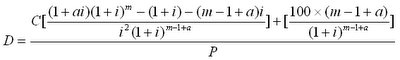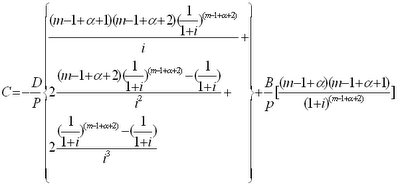• This service has been inactive since 2010 as my new role in TM1 consulting.
• Please disregard any commercial offers as this website has been archived and transformed into a blog rather than a commercial website.

Bond Price, Duration and ConvexityIn Feb 06 I submitted some short of article in Wikipedia about closed form formulas for bond duration and convexity. Below is the clone and hopefully it can be useful for those who may use the formulas for bond analysis modelling in Excel spreadsheets.

As far as I know, these have never been published on the web like this as I experienced some problem in finding them when doing some bond pricing project.

Closed-form formula:
A single arithmetic formula obtained to simplify an infinite sum in a general formula. The general formula of bond duration and bond convexity cannot be said closed-form as there is an infinite sum over the different time periods. Using a closed-form formula, a bond’s duration or convexity can be calculated at any point in its life time.

Bond duration closed-form formula (Richard Klotz):C = coupon payment per period (half-year)
P = present value (price)
i = discount rate per period (half-year)
a = fraction of a period remaining until next coupon payment
m = number of coupon dates until maturity

Bond convexity closed-form formula (Blake and Orszag):D = coupon payment per period
P = present value (price)
B = face value
i = discount rate per period (half-year)
a = fraction of a period remaining until next coupon payment
m = number of coupon dates until maturity